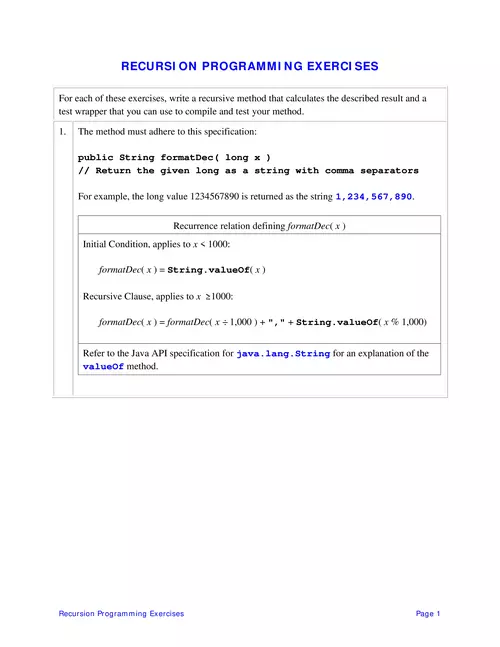### RECURSION PROGRAMMING EXERCISES

Refer to the Java API specification for java.lang.String for an explanation of the. valueOf method. Page 2. Recursion Programming

#### Part of the document

Recursion Programming ExercisesPage1
RECURSION PROGRAMMING EXERCISESFor each of these exercises, write a recursive method that calculates the described result and atest wrapper that you can use to compile and test your method.1.The method must adhere to this specification:public String formatDec( long x )// Return the given long as a string with comma separatorsFor example, the long value 1234567890 is returned as the string1,234,567,890.Recurrence relation definingformatDec(x)Initial Condition, applies tox< 1000:formatDec(x) =String.valueOf(x)Recursive Clause, applies tox≥ 1000:formatDec(x) =formatDec(x÷1,000 ) +","+String.valueOf(x% 1,000)Referto the Java API specification forjava.lang.Stringfor an explanation of thevalueOfmethod.
Recursion Programming ExercisesPage2
2.The following table shows how a Certificate of Deposit grows in value each period ofinvestment. The table assumes the CD starts with \$1,000, the APR (annual percentage rate)is 5% and the CD"s interest is compounded monthly (i.e. the period is 1 month).As shown in the table, interest "compounding" is accomplished by applying the interest rateto the total of the deposits plus interest.Recurrence relation defining CD(n) = the value of the CD afternperiodsInitial Condition:CD( 0 ) =initial depositRecursive Clause:CD(n) = ( 1 +period rate) × CD(n- 1 )initial depositis \$1,000 in the example above.period rate= APR ÷ 100 ÷number of periods per year. The example above has amonthly compounding period so the number of periods per year is 12 and theperiod rateis 5/100/12.Your method must adhere to this specification:public double cd( int n, double d, double apr, int period )// Return the value of a CD after n periods.// d = initial deposit,// apr = annual percentage rate// period = number of periods per year
Recursion Programming ExercisesPage3
3.The method must adhereto this specification:public String reverse( String s )// Return the reverse of the given string.Recurrence relation definingreverse(s)Initial Condition:reverse(null string) =null stringRecursive Clause:reverse(s) =reverse(s.substring(1))+s.charAt( 0 )Referto the Java API specification forjava.lang.Stringfor explanations of thecharAtandsubstringmethods.